﻿ 一种提高SVM分类能力的同步优化算法 A Synchronous Optimization Algorithm for Increasing Accuracy of SVM Classification

Vol.06 No.09(2017), Article ID:23028,9 pages
10.12677/AAM.2017.69130

A Synchronous Optimization Algorithm for Increasing Accuracy of SVM Classification

Fan He, Changjing Lu

School of Mathematics and Physics, China University of Geosciences, Wuhan HubeiReceived: Nov. 24th, 2017; accepted: Dec. 7th, 2017; published: Dec. 14th, 2017ABSTRACT

Support vector machines (SVM), which is a popular method for pattern classification, has been recently adopted in range of problems. In training procedure of SVM, feature selection and parameter optimization are two main factors that impact on classification accuracy. In order to improve the classification accuracy by optimizing parameter and choosing feature subset for SVM, a new algorithm is proposed through combining Bat Algorithm (BA) with SVM, termed BA + SVM. For assessing the performance of BA + SVM, 10 public data-sets are employed to test the classification accuracy rate. Compared with grid algorithm, conventional parameter optimization method, our study concludes that BA + SVM has a higher classification accuracy with fewer input features for support vector classification.

Keywords:SVM, Bat Algorithm, Feature Selection, Parameter Optimization, Classification1. 引言

2. 相关工作

2.1. SVM分类器

$〈\omega \cdot {x}_{i}〉+b=0,\text{\hspace{0.17em}}\text{\hspace{0.17em}}i=1,2,\cdots ,m$ (1)

$\begin{array}{l}\underset{1\le i\le m}{\mathrm{min}}\frac{1}{2}{\omega }^{\text{T}}\omega +C\underset{i=1}{\overset{m}{\sum }}{\xi }_{i}\\ \text{subjectto}:{y}_{i}\left(〈\omega \cdot {x}_{i}〉+b\right)-1\ge 0\end{array}$ (2)

$f\left(x\right)=sign\left(\underset{i=1}{\overset{m}{\sum }}{y}_{i}{\alpha }_{i}^{*}〈{x}_{i}\cdot x〉+{b}^{*}\right)$ (3)

$f\left(x,{\alpha }_{i}^{*},{b}^{*}\right)=sign\left(\underset{i=1}{\overset{m}{\sum }}{y}_{i}{\alpha }_{i}^{*}k\left({x}_{i},x\right)+{b}^{*}\right)$ (4)

2.2. 蝙蝠算法

${f}_{i}={f}_{\mathrm{min}}+\left({f}_{\mathrm{max}}-{f}_{\mathrm{min}}\right)\beta$ (5)

${v}_{i}^{t+1}={v}_{i}^{t}+\left({x}_{i}^{t}-{x}_{*}\right){f}_{i}$ (6)

${x}_{i}^{t+1}={x}_{i}^{t}+{v}_{i}^{t+1}$ (7)

${x}_{new}={x}_{old}+\epsilon {A}^{t}$ (8)

${A}_{i}^{t+1}=\alpha {A}_{i}^{t}$ (9)

${r}_{i}^{t+1}={r}_{i}^{0}\left[1-\mathrm{exp}\left(-\gamma t\right)\right]$ (10)

2.3. 特征选择

3. 基于BA的SVM特征选择和参数优化

3.1. 蝙蝠位置的表示

3.2. 蝙蝠位置的更新标准

3.3. 适应度函数

$fi{t}_{i}={\omega }_{A}\cdot ac{c}_{i}+{\omega }_{F}\cdot \left(1-\frac{\underset{j=1}{\overset{n}{\sum }}{f}_{j}}{n}\right)$ (11)

${\omega }_{A}$ 是SVM的分类准确率权重， ${\omega }_{F}$ 是所选特征数量的权重，用户可根据需要进行适当调整。如果选择了特征j， ${f}_{j}=1$ ，否则 ${f}_{j}=0$$ac{c}_{i}$ 表示SVM分类准确率，由公式(12)给出。 $ac$$uc$ 分别表示正确分类的样本数和不正确分类的样本数。

$ac{c}_{i}=\frac{cc}{cc+uc}×100%$ (12)

4. BA + SVM参数优化和特征选择算法

BA + SVM参数优化和特征选择的流程图如图1所示，详细的实验步骤如下：Table 1. The composition of the location of the bat i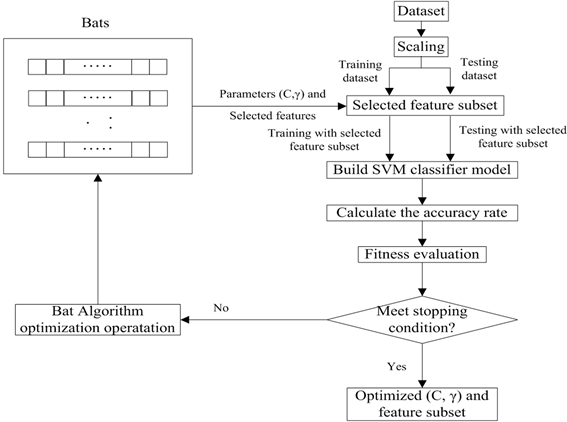Figure 1. Flow chart: BA + SVM parameter optimization and feature selection

5. 实验结果

5.1. 平台和数据集

5.2. 评估方法Table 3. Classification of the two categories of issues

TP和FN分别表示正样本的正确分类率和正样本的不正确分类率，是两个重要的性能指标，计算公式表示如下：

$\text{TP}=\frac{#\text{TruePositive}}{#\text{FalseNegative}+#\text{TruePositive}}$ (13)

$\text{TN}=\frac{#\text{TureNegative}}{#\text{TrueNegative}+#\text{FalsePositive}}$ (14)

$\text{Averageaccuracy}=\frac{#\text{TruePositive}+#\text{TruePositive}}{#\text{TestingSample}}$ (15)

5.3. 实验结果Table 5. Comparison of classification results between BA + SVM and SVM and PSO + SVMTable 6. Comparison of BA optimization and grid optimization without feature selection

6. 结论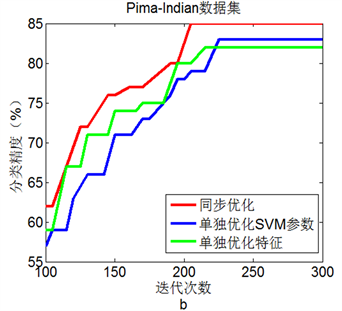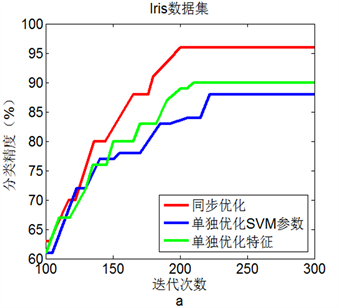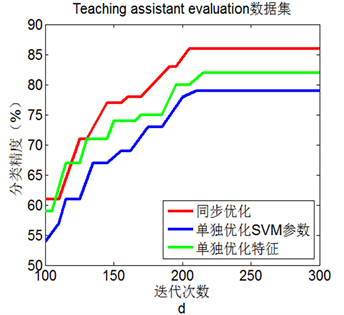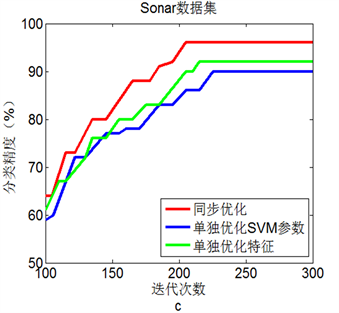Figure 2. Line chart: Comparison of three experimental results on 4 data sets

A Synchronous Optimization Algorithm for Increasing Accuracy of SVM Classification[J]. 应用数学进展, 2017, 06(09): 1073-1081. http://dx.doi.org/10.12677/AAM.2017.69130

1. 1. Vapnik, V.N. (1995) The Nature of Statistical Learning Theory. Springer-Verlag, New York.
https://doi.org/10.1007/978-1-4757-2440-0

2. 2. Joachims, T. (1998) Text Categorization with Support Vector Machines: Learning with Many Relevant Features. Proceedings of Support Vector Learning Machine Learning: ECML-98, Volume 1398 of the Series Lecture Notes in Computer Science, 137-142.
https://doi.org/10.1007/BFb0026683

3. 3. Yu, G.-X., Ostrouchov, G., Geist, A., et al. (2003) An SVM Based Algorithm for Identification of Photosynthesis-Specific Genome Features. Proceedings of the 2003 IEEE Bioinformatics Conference on Computational Systems Bioinformatics, California, 235-243.
https://doi.org/10.1109/CSB.2003.1227323

4. 4. Keerthi, S.S. and Lin, C.-J. (2003) Asymptotic Behaviors of Support Vector Machines with Gaussian Kernel. Neural Computation, 15, 1667-1689.
https://doi.org/10.1162/089976603321891855

5. 5. Xiao, H.J., Wei, Y.B., Yu, Z.L., et al. (2010) A New Active Learning Method for Instance Selection. Journal of Information & Computational Science, 7, 2789-2795.

6. 6. Oliveira, A.L.I., Braga, P.L., Lima, R.M.F. and Cornélio, M.L. (2010) GA-Based Method for Feature Selection and Parameters Optimization for Machine Learning Regression Applied to Software Effort Estimation. Information and Software Technology, 52, 1155-1166.
https://doi.org/10.1016/j.infsof.2010.05.009

7. 7. Huang, C.-L. and Dun, J.-F. (2008) A Distributed PSO-SVM Hybrid System with Feature Selection and Parameter Optimization. Applied Soft Computing, 8, 1381-1391.
https://doi.org/10.1016/j.asoc.2007.10.007

8. 8. Lin, S.W., Ying, K.C., Chen, S.C., et al. (2008) Particle Swarm Optimization for Parameter Determination and Feature Selection of Support Vector Machines. Expert Systems with Applications, 35, 1817-1824.
https://doi.org/10.1016/j.eswa.2007.08.088

9. 9. Li, X.F. (2014) Network Intrusion Detection with Genetic Algo-rithm Synchronous Selecting Feature and SVM Parameters. Computer Applications and Software, 63, S76.

10. 10. Griffin, D.R., Webster, F.A., Michael, C.R., et al. (1960) The Echolocation of Flying Insects by Bats. Animal Behaviour, 8, 141-154.
https://doi.org/10.1016/0003-3472(60)90022-1

11. 11. Tharwat, A., Hassanien, A.E. and Elnaghi, B.E. (2017) A BA-Based Algorithm for Parameter Optimization of Support Vector Machine. Pattern Recognition Letters, 93, 13-22.
https://doi.org/10.1016/j.patrec.2016.10.007

12. 12. Metzner, W. (1991) Echolocation Behaviour in Bats. Science Progress Edinburgh, 75, 453-465.

13. 13. Liu, H. and Motoda, H. (1998) Feature Selection for Knowledge Discovery and Data Mining. Kluwer Academic, Boston.

14. 14. Chen, R.-C. and Hsieh, C.-H. (2006) Web Page Classification Based on a Support Vector Machine Using a Weighed Vote Schema. Expert Systems with Applications, 31, 427-435.
https://doi.org/10.1016/j.eswa.2005.09.079

15. 15. Chang, C.C. and Lin, C.J. (2001) LIBSVM: A Library for Support Vector Machines. http://www.csie.ntu.edu.tw/~cjlin/libsvm